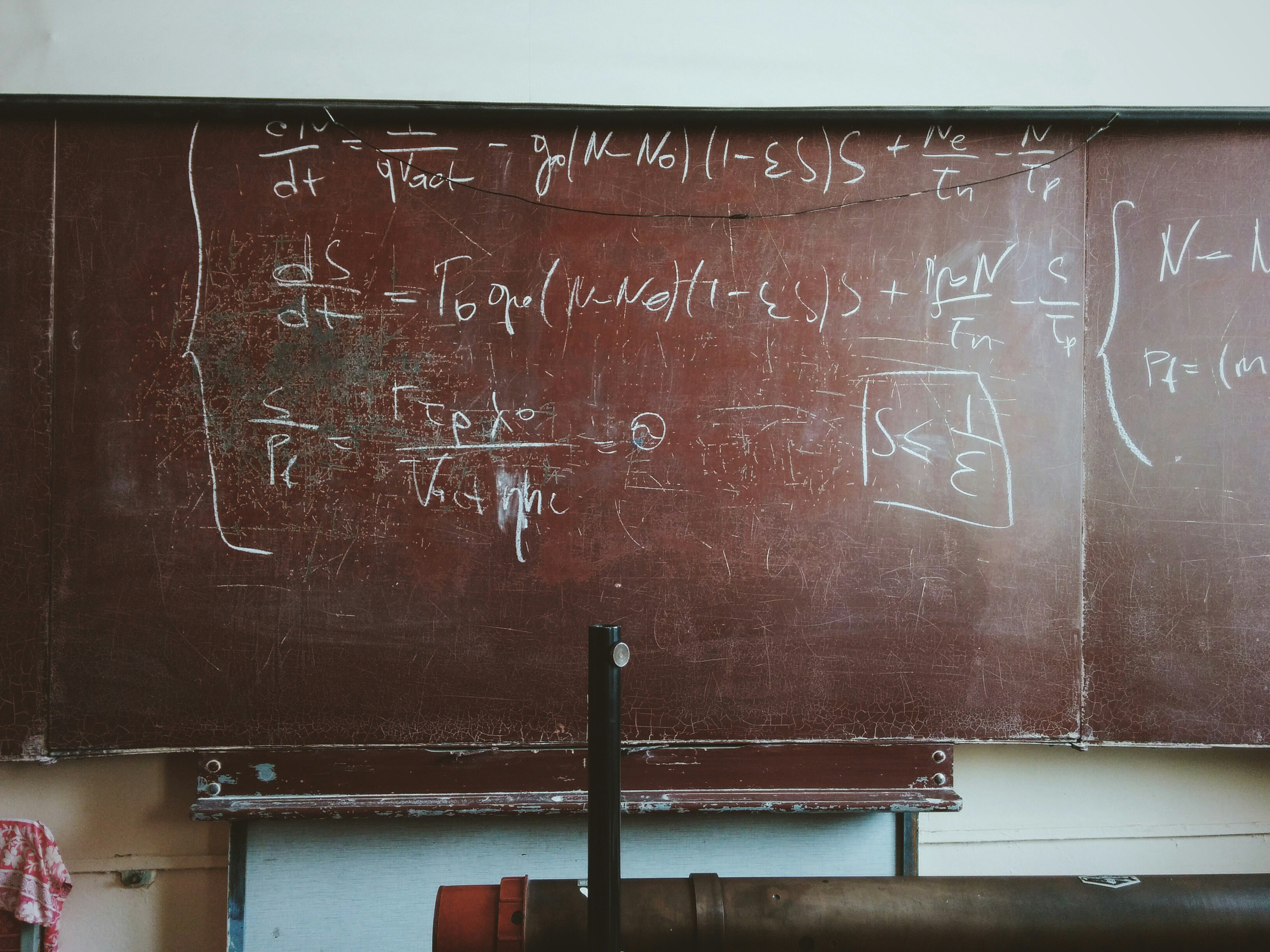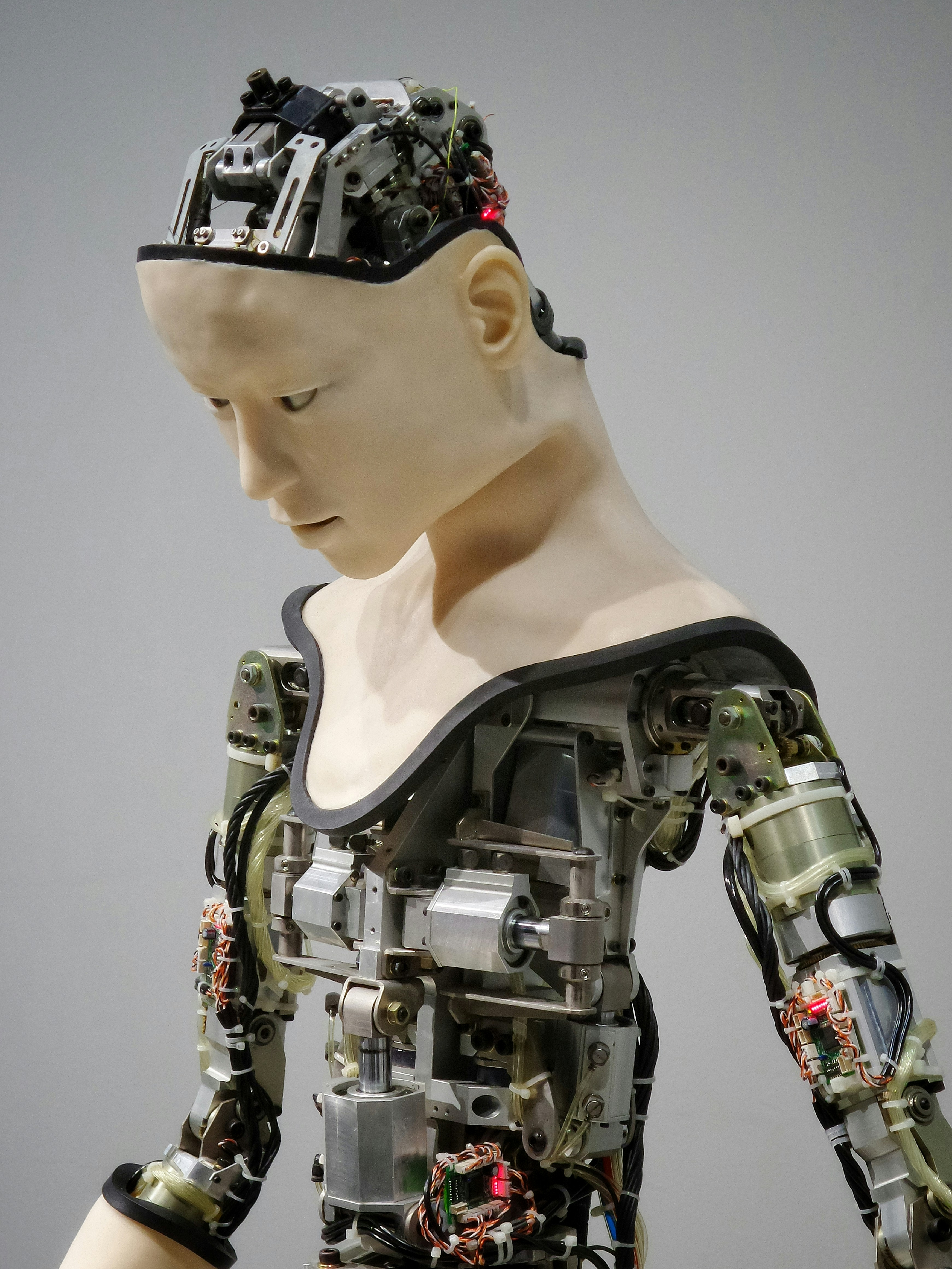## Hello

Latest ArticlesFinal Laboratory Assignment: Using K-Nearest Neighbors, Apriori, and Decision Tree j48 Introduction The dataset I’ve decided to explore is analyzing red wine dataset to determine the quality. There is a total of 1599 instances and 12 elements that determine…Introduction Note: This is a living document for documentation purposes, and I will be making changes as I go. I will be building this tool in Python using the Flask framework. This is a pretty simple application, and I wanted…Starting at the basic level, machine learning is a set of tasks working together to solve a problem. It is also a subfield of Artificial Intelligence. Machine learning uses a combination of statistical analysis, mathematics such as linear algebra, and…